# How to Only Return Results from Non-Blank Cells in Microsoft Excel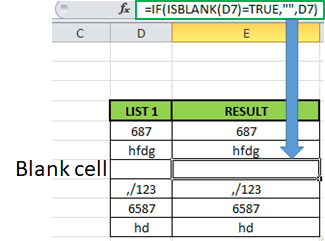In this article, we will learn How to Only Return Results from Non-Blank Cells in Microsoft Excel.

What is a blank and non blank cell in Excel?

In Excel, Sometimes we don't even want blank cells to disturb the formula. Most of the time we don't want to work with a blank cell as it creates errors using the function. For Example VLOOKUP function returns #NA error when match type value is left blank. There are many functions like ISERROR which treats errors, but In this case we know the error is because of an empty value. So We learn the ISBLANK function in a formula with IF function.

IF - ISBLANK formula in Excel

ISBLANK function returns TRUE or FALSE boolean values based on the condition that the cell reference used is blank or not. Then use the required formula in the second or third argument.

Syntax of formula:

 =IF(ISBLANK(cell_ref),[formula_if_blank],[ formula_if_false]

Cell ref : cell to check, if cell is blank or not.

Formula_if_true : formula or value if the cell is blank. Use Empty value ("") if you want empty cell in return

Formula_if_false : formula or value if the cell is not blank. Use Empty value ("") if you want empty cell in return

Example :

All of these might be confusing to understand. Let's understand how to use the function using an example. Here we have some values on list 1 and we only want the formula to return the same value if not blank and empty value if blank.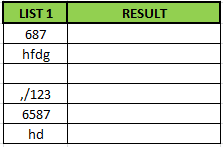Formula:

 =IF(ISBLANK(D5)=TRUE,"", D5)

Explanation:

ISBLANK : function checks the cell D5.

"" :  returns an empty string blank cell instead of Zero.

IF function performs a logic_test if the test is true, it returns an empty string else returns the same value.

The resulting output will be like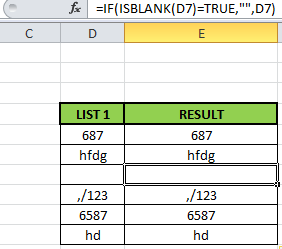As you can see the formula returns the same value or blank cell based on the logic_test.

ISBLANK function with example

ISBLANK function returns TRUE or FALSE having cell reference as input.

Syntax:

 =ISBLANK (cell_ref)

Cell_ref : cell reference of cell to check e.g. B3

All of these might be confusing to understand. Let's understand how to use the function using an example. Here We have some Values to test the function, except in the A5 cell. A5 cell has a formula that returns empty text.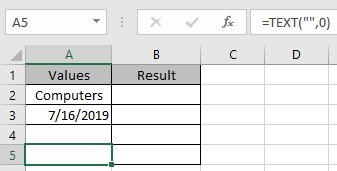Use the Formula

 =ISBLANK(A2)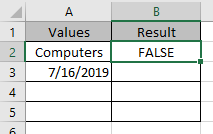It returns False as there is text in the A2 cell.

Applying the formula in other cells using Ctrl + D shortcut key.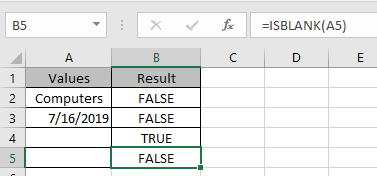You must be wondering why didn’t the function returns False. As A5 cell has a formula making it non blank.

Hope this article about How to Only Return Results from Non-Blank Cells in Microsoft Excel is explanatory. Find more articles on blank values and related Excel formulas here. If you liked our blogs, share it with your friends on Facebook. And also you can follow us on Twitter and Facebook. We would love to hear from you, do let us know how we can improve, complement or innovate our work and make it better for you. Write to us at info@exceltip.com.

Related Articles :

Adjusting a Formula to Return a Blank : Formula adjusts its result on the basis of whether the cell is blank or not. Click the link to know how to use ISBLANK function with IF function in Excel.

Checking Whether Cells in a Range are Blank, and Counting the Blank Cells : If cells in a range are blank and calculating the blank cells in Excel then follow the link to understand more.

How to Calculate Only If Cell is Not Blank in Excel : Calculate only and only if the cell is not blank then use ISBLANK function in Excel.

Delete drop down list in Excel : The dropdown list is used to restrict the user to input data and gives the option to select from the list. We need to delete or remove the Dropdown list as the user will be able to input any data instead of choosing from a list.

Deleting All Cell Comments in Excel 2016 : Comments in Excel to remind ourselves and inform someone else about what the cell contains. To add the comment in a cell, Excel 2016 provides the insert Comment function. After inserting the comment it is displayed with little red triangles.

How to Delete only Filtered Rows without the Hidden Rows in Excel :Many of you are asking how to delete the selected rows without disturbing the other rows. We will use the Find & Select option in Excel.

How to Delete Sheets Without Confirmation Prompts Using VBA In Excel :There are times when we have to create or add sheets and later on we find no use of that sheet, hence we get the need to delete sheets quickly from the workbook

Popular Articles :

How to use the IF Function in Excel : The IF statement in Excel checks the condition and returns a specific value if the condition is TRUE or returns another specific value if FALSE.

How to use the VLOOKUP Function in Excel : This is one of the most used and popular functions of excel that is used to lookup value from different ranges and sheets.

How to use the SUMIF Function in Excel : This is another dashboard essential function. This helps you sum up values on specific conditions.

How to use the COUNTIF Function in Excel : Count values with conditions using this amazing function. You don't need to filter your data to count specific values. Countif function is essential to prepare your dashboard.

1.?????????? ????
_________________
????? ?? ??????????? ?????? 777

2.Hi can someone please help. I want a cell to have today's date in, TODAY(), only if another cell is completed. So we only need today's date if a start date has been entered already. Can someone help me with the formular. Many thanks

•Hi Donna,
Let's say you put the start date in A2 and you want today's date in B2 then use this formula in B2.
=IF(ISBLANK(A2),"",TODAY())

3.Good Morning. I am trying to use Conditional Formatting with the ISBLANK.
What I am after is if there is a Value in any CELL eg. (S2:T501), Format the Corresponding Cell in F. eg if there is a Value in S14, then F14 will be formatted. Or Do I have to Conditionally Format each Cell in Col F
Thanking you

4.I have a column of times with variable blank cells between them. Columns are unit numbers.

I want to sort the times automatically with no blank cells between them in new cells so I can use a forumla that to figures out average trip times per unit ie start time and end time

5.Hi, how would I write a formula if it contains 2 x IF's?

I.e. If Cell Q2 is not blank, then calculate IF(Q2>TODAY(), "VALID", "EXPIRED"

6.I have a spreadsheet where a due date in column H and date mailed in Column I. i have a calculation in column J to see if we met the 15 day requirement yes or no: =IF(AND(I2<=H2),"Yes","No").
Problem is its displaying yes in all the fields that don't even have a due date yet. How can I get this not to show anything if a there is no due date entered?

7.The following formula is not working for me =IF(ISBLANK(B4)=FALSE,DAY(B4),””)

Here B4 contain a Date. The out put is #NAME?

My requirement is needs a blank cell instead of 0 in other cell if the B4 cell is blank.

8.Dan Akers' formula was a big help to me. I have a running list and wanted to auto-number each line as new items were added (ie, when something is typed into a field on row 7, another cell on that row would auto-enter a "7" to keep track of how many lines are populated). It's a stupid calculation but I didn't want to drag out 1000 numbers for empty rows. =IF(ISBLANK(B82)=FALSE,(A81+1),””) worked like a champ. Thanks again.

9.I have a date in Column A, and I have a formula in Column H as follows: =SUM(A3+30)
So a date appears in Column H which is 30 days after the date in Column A. Problem is that if there is no date in Column A, this appears in Column H: #VALUE! Is there any way to prevent this? I've spent days trying to figure this out. Greatly appreciated!!!!

•Try this formula in H3, assuming A3 is the source date.
=IF(ISBLANK(A3)=FALSE,(A3+30),””)

•The following formula is not working for me =IF(ISBLANK(B4)=FALSE,DAY(B4),””)

Here B4 contain a Date. The out put is #NAME?

My requirement is needs a blank cell instead of 0 in other cell if the B4 cell is blank.

•Thanks, Dan! worked perfectly!!!

•Thanks Dan!

Terms and Conditions of use

The applications/code on this site are distributed as is and without warranties or liability. In no event shall the owner of the copyrights, or the authors of the applications/code be liable for any loss of profit, any problems or any damage resulting from the use or evaluation of the applications/code.